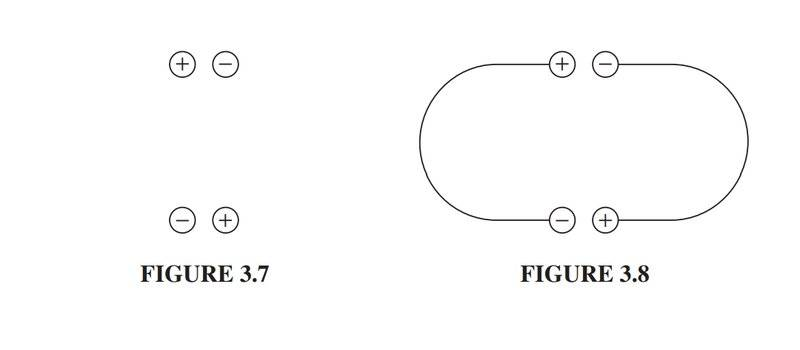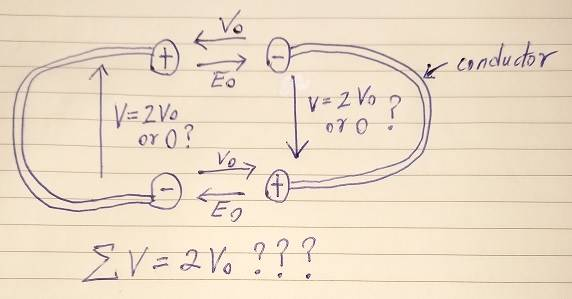# A question about the Second Uniqueness Theorem in electrostatics

• I
Ahmed1029in this example in Griffiths' electrodynamics, he says the following :

(Figure 3.7 shows
a simple electrostatic configuration, consisting of four conductors with charges
±Q, situated so that the plusses are near the minuses. It all looks very comfort-
able. Now, what happens if we join them in pairs, by tiny wires, as indicated in
Fig. 3.8? Since the positive charges are very near negative charges (which is where
they like to be) you might well guess that nothing will happen—the configuration
looks stable.
Well, that sounds reasonable, but it’s wrong. The configuration in Fig. 3.8 is
impossible. For there are now effectively two conductors, and the total charge
on each is zero. One possible way to distribute zero charge over these conductors is to have no accumulation of charge anywhere, and hence zero field everywhere)

I feel like there are gaps in this explanation, that is, don't know if he implicitly invoked the first uniqueness theorem here

•Delta2

Homework Helper
This is like connecting two oppositely charged capacitors. You bet the current will flow!

•Delta2
Homework Helper
Gold Member
Well he seems to imply that since one way to redistribute zero total charge over a conductor, is to have zero charge everywhere in the conductor, then this is the only way. Is this the first uniqueness theorem btw?

Ahmed1029
Well he seems to imply that since one way to redistribute zero total charge over a conductor, is to have zero charge everywhere in the conductor, then this is the only way. Is this the first uniqueness theorem btw?
Well there are infinite ways to distribute charge on conductors, each with its own electric field. What conditions will tell me which of them is the true one? This is the second uniqueness theorem

Homework Helper
A conductor has no potential difference. The charge may be distributed unevenly, but the surface potential is the same everywhere.

##\ ##

•vanhees71
alan123hk
I feel like there are gaps in this explanation, that is, don't know if he implicitly invoked the first uniqueness theorem here
The situation shown in Figure 3.8 is a fictitious assumption and it is likely not a stable state.

If we think of these two conductors as an isolated capacitor, there is no potential difference and no electric field between them since the net charge in the two conductors is zero. That is, there is also no charge accumulation on the surfaces of the two conductors.

Homework Helper
Gold Member
Hmm, given a conductor (that is given its shape) and a total charge Q, isn't the way that this charge Q going to redistribute itself along the surface of the conductor, unique? And that depends only on the shape of the conductor?

•vanhees71
alan123hk
Hmm, given a conductor (that is given its shape) and a total charge Q, isn't the way that this charge Q going to redistribute itself along the surface of the conductor, unique? And that depends only on the shape of the conductor?
I think so too, which seems to be what the uniqueness theorem says.

•vanhees71 and Delta2
Homework Helper
Hmm, given a conductor (that is given its shape) and a total charge Q, isn't the way that this charge Q going to redistribute itself along the surface of the conductor, unique? And that depends only on the shape of the conductor?

There is the small matter of an external field (if present)

##\ ##

•vanhees71 and Delta2
Homework Helper
Gold Member
Yes that's very right, I should 've said in the presence of no external field.

alan123hk
In fact, the situation of Figure 3.8 is impossible because it violates the conservation of energy around closed circuit paths and circuit theory.•Delta2
Homework Helper
Gold Member
Yes we can't process this with circuit theory, unless the conductors have some ohmic resistance.

•alan123hk